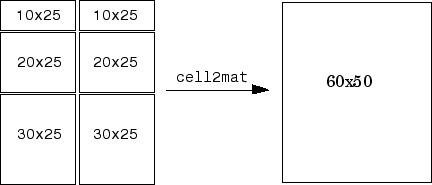# cell2mat

Convert cell array to ordinary array of the underlying data type

## Syntax

``A = cell2mat(C)``

## Description

example

````A = cell2mat(C)` converts a cell array into an ordinary array. The elements of the cell array must all contain the same data type, and the resulting array is of that data type.The contents of `C` must support concatenation into an N-dimensional rectangle. Otherwise, the results are undefined. For example, the contents of cells in the same column must have the same number of columns, although they need not have the same number of rows (see figure).```

## Examples

collapse all

Convert numeric arrays in four cells of a cell array into one numeric array.

```C = {, [2 3 4]; [5; 9], [6 7 8; 10 11 12]}```
```C=2×2 cell array {[ 1]} {[ 2 3 4]} {2x1 double} {2x3 double} ```
`A = cell2mat(C)`
```A = 3×4 1 2 3 4 5 6 7 8 9 10 11 12 ```

Convert structures in a cell array into one structure array. The structures must have the same fields.

```s1.a = [1 2 3 4]; s1.b = 'Good'; s2.a = [5 6; 7 8]; s2.b = 'Morning'; c = {s1,s2}; d = cell2mat(c)```
```d=1×2 struct array with fields: a b ```

Display the first field of structure `d(1)`.

`d(1).a`
```ans = 1×4 1 2 3 4 ```

Display the second field of `d(2)`.

`d(2).b`
```ans = 'Morning' ```

## Input Arguments

collapse all

Input cell array, in which all cells contain the same data type. `cell2mat` accepts numeric, logical, or character data within cells of `C`, or structures with the same field names and data types. `cell2mat` does not accept objects or nested cells within `C`.

## Version History

Introduced before R2006a